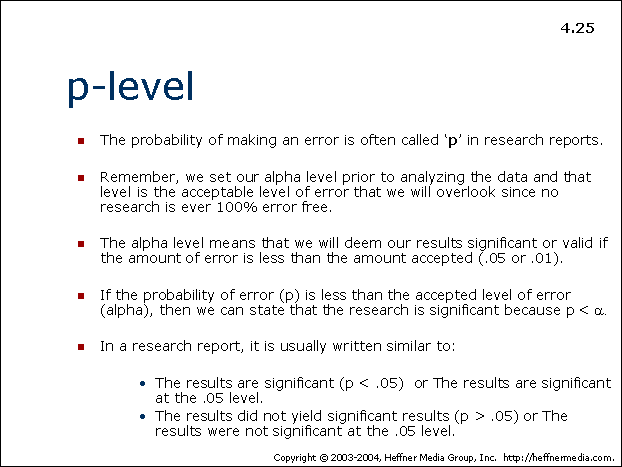# 25: p-level## p-level

• The probability of making an error is often called ‘p’ in research reports.
• Remember, we set our alpha level prior to analyzing the data and that level is the acceptable level of error that we will overlook since no research is ever 100% error free.
• The alpha level means that we will deem our results significant or valid if the amount of error is less than the amount accepted (.05 or .01).
• If the probability of error (p) is less than the accepted level of error (alpha), then we can state that the research is significant because p < α.
• In a research report, it is usually written similar to:
• The results are significant (p < .05) or The results are significant at the .05 level.
• The results did not yield significant results (p > .05) or The results were not significant at the .05 level.